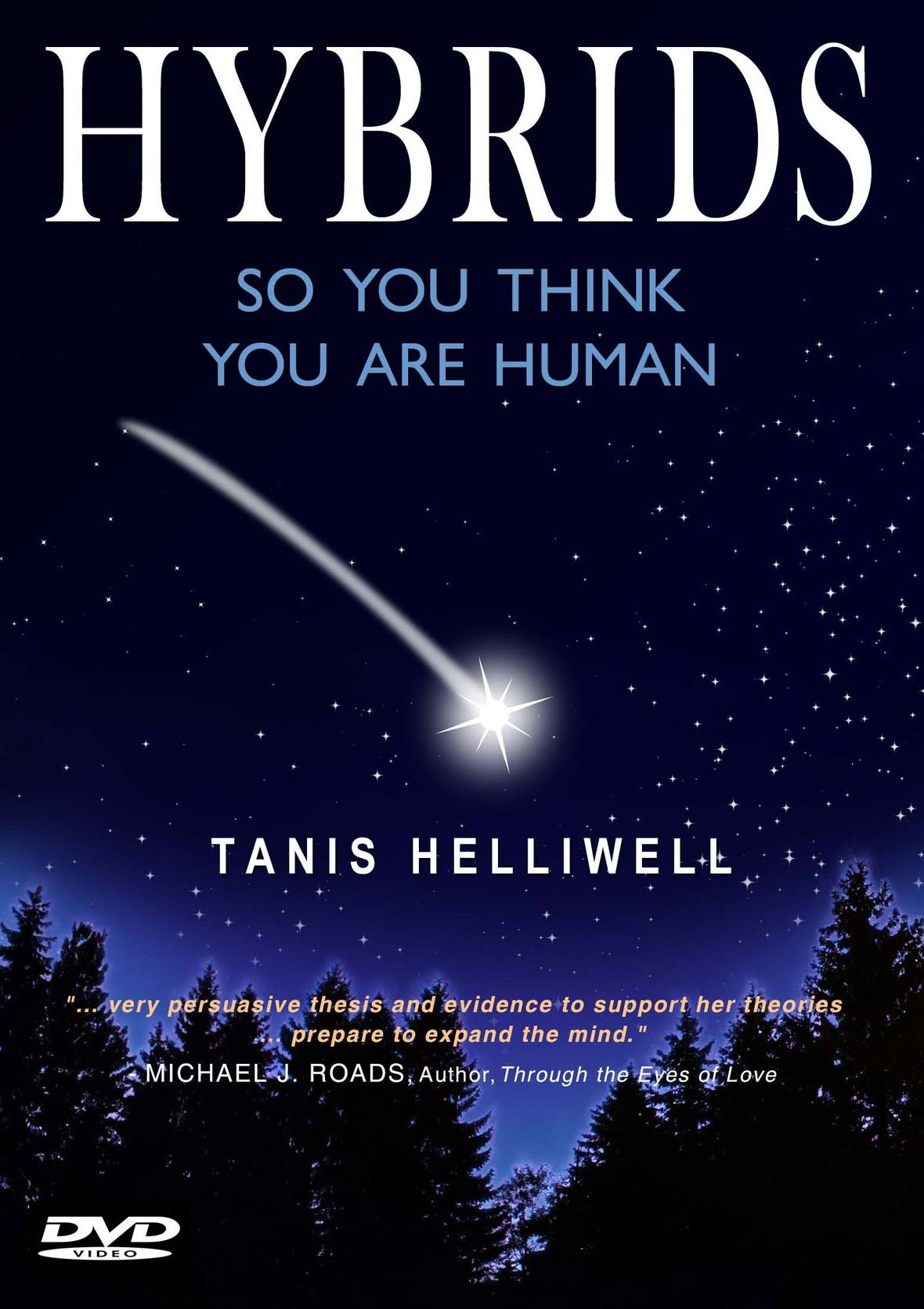# NOVEMBER 2018

## Happy Thanksgiving to my American friends!Enjoy your Thanksgiving dinner later this month -- I do hope you get to indulge in some REAL pumpkin pie!

Dear Friend,

▪ A brand new Youtube video talk about how I came to work with human-hybrids. The video was recorded at a workshop I gave recently in Freiburg, Germany.
▪ For the month of November, I'm offering a 10% discount on my newest book and DVD, HYBRIDS: So you think you are human
▪ Want a preview of my Hybrids DVD? See my introductory video below!
 ▪ A brand new Youtube video talk about how I came to work with human-hybrids. The video was recorded at a workshop I gave recently in Freiburg, Germany.
 ▪ For the month of November, I'm offering a 10% discount on my newest book and DVD, HYBRIDS: So you think you are human
 ▪ Want a preview of my Hybrids DVD? See my introductory video below!
 table div table+table+table+table+table div table{width:100%;padding:0}table div table+table+table+table+table div table img{width:96.23%;padding:0;float:none}table div table+table+table+table+table div table td{width:100%;padding:0 1.88% 18px}/* styles *//* styles */
 table div table+table+table+table+table+table+table div table{width:100%;padding:0}table div table+table+table+table+table+table+table div table img{width:96.23%;padding:0;float:none}table div table+table+table+table+table+table+table div table td{width:100%;padding:0 1.88% 18px}/* styles */## NEW VIDEO: What are human-hybrids?

 /* styles */ Over the past two years, I have been asked to do many workshops and presentations on my research and experiences with human-hybrids. I have published a book and a DVD on the topic, but in my workshops I also discuss in more detail how I came to learn of the existence of these beings and how this knowledge can benefit us all in our everyday lives. The video below was recorded at one of these sessions in Freiburg, Germany and runs just over 26 minutes. Many thanks as always to Monika Bernegg for the simultaneous German translation. Enjoy! (Click on the image below to view the video, or link directly here: https://youtu.be/J8X8Z4akR2w)
 table div table+table+table+table+table+table+table+table+table+table div table td,table.module-9{width:100%;padding:0}table div table+table+table+table+table+table+table+table+table+table div table{width:100%;float:none;margin-left:auto;margin-right:auto;padding:0}table div table+table+table+table+table+table+table+table+table+table div table a{border:0 none;text-decoration:none}table div table+table+table+table+table+table+table+table+table+table div table img{width:100%!important;border:0 none;text-decoration:none}/* styles */
 table div table+table+table+table+table+table+table+table+table+table+table div table{width:100%;padding:0}table div table+table+table+table+table+table+table+table+table+table+table div table img{width:96.23%;padding:0;float:none}table div table+table+table+table+table+table+table+table+table+table+table div table td{width:100%;padding:0 1.88% 18px}/* styles */# Paperback & Kindle

Myths, religions and archaeological discoveries around the world discuss the presence of intelligent non-human beings who have interbred with humans creating hybrids. People who discover they are hybrids report feeling more authentically themselves—more energized, powerful and self-loving—as a result of their discovery.

Am I a Hybrid?

If you answer “yes” to these statements, Hybrids: So you think you are human will be of special interest to you:

▪ I often feel I don’t fit in
▪ I am hypersensitive to violence
▪ I am deeply committed to helping the Earth
▪ I would describe myself as an independent thinker
▪ I follow my own inner guidance about rightness, even if it goes against the norm
 ▪ I often feel I don’t fit in
 ▪ I am hypersensitive to violence
 ▪ I am deeply committed to helping the Earth
 ▪ I would describe myself as an independent thinker
 ▪ I follow my own inner guidance about rightness, even if it goes against the norm

As with her ground-breaking work on elementals in the classic Summer with the Leprechauns, Tanis is, with Hybrids, once again at the forefront of an entire new genre of investigation.

Chapters include:

▪ What is a Hybrid?
▪ How do we know that Hybrids exist?
▪ What type of Elemental Hybrid am I?
▪ Or, What type of Human Cousin Hybrid am I?
▪ Or, What type of Star Being Hybrid am I?
 ▪ What is a Hybrid?
 ▪ How do we know that Hybrids exist?
 ▪ What type of Elemental Hybrid am I?
 ▪ Or, What type of Human Cousin Hybrid am I?
 ▪ Or, What type of Star Being Hybrid am I?

The book also includes a detailed questionnaire to help you determine what type of hybrid you might be!

## PAPERBACK is only \$13.50 for November, and KINDLE is only \$8.95

 table div table+table+table+table+table+table+table+table+table+table+table+table+table+table+table+table div table{width:100%;padding:0}table div table+table+table+table+table+table+table+table+table+table+table+table+table+table+table+table div table img{width:96.23%;padding:0;float:none}table div table+table+table+table+table+table+table+table+table+table+table+table+table+table+table+table div table td{width:100%;padding:0 1.88% 18px}/* styles */# DVD format▪ 45 minutes (see below for a free preview!)
 ▪ 45 minutes (see below for a free preview!)

The DVD Hybrids: So you think you are human is a wonderful companion to Tanis’ book of the same title (see above).

Tanis Helliwell offers convincing evidence in this DVD about the existence of 22 different human-hybrids (elementals, angels, dolphins, giants, dragons, centaurs, pan and many others).

Only \$13.45 for the month of November

 table div table+table+table+table+table+table+table+table+table+table+table+table+table+table+table+table+table+table+table+table div table{width:100%;padding:0}table div table+table+table+table+table+table+table+table+table+table+table+table+table+table+table+table+table+table+table+table div table img{width:96.23%;padding:0;float:none}table div table+table+table+table+table+table+table+table+table+table+table+table+table+table+table+table+table+table+table+table div table td{width:100%;padding:0 1.88% 18px}/* styles */# DVD Preview:

Click on the image below to view this Youtube video introduction to the DVD, or use the direct link here: https://youtu.be/ZpoLBfr33hs

 table div table+table+table+table+table+table+table+table+table+table+table+table+table+table+table+table+table+table+table+table+table+table div table td,table.module-21{width:100%;padding:0}table div table+table+table+table+table+table+table+table+table+table+table+table+table+table+table+table+table+table+table+table+table+table div table{width:100%;float:none;margin-left:auto;margin-right:auto;padding:0}table div table+table+table+table+table+table+table+table+table+table+table+table+table+table+table+table+table+table+table+table+table+table div table a{border:0 none;text-decoration:none}table div table+table+table+table+table+table+table+table+table+table+table+table+table+table+table+table+table+table+table+table+table+table div table img{width:100%!important;border:0 none;text-decoration:none}/* styles */
 table div table+table+table+table+table+table+table+table+table+table+table+table+table+table+table+table+table+table+table+table+table+table+table div table{width:100%;padding:0}table div table+table+table+table+table+table+table+table+table+table+table+table+table+table+table+table+table+table+table+table+table+table+table div table img{width:96.23%;padding:0;float:none}table div table+table+table+table+table+table+table+table+table+table+table+table+table+table+table+table+table+table+table+table+table+table+table div table td{width:100%;padding:0 1.88% 18px}/* styles */table div table+table+table+table+table+table+table+table+table+table+table+table+table+table+table+table+table+table+table+table+table+table+table+table+table div table{width:100%;padding:0}table div table+table+table+table+table+table+table+table+table+table+table+table+table+table+table+table+table+table+table+table+table+table+table+table+table div table img{width:96.23%;padding:0;float:none}table div table+table+table+table+table+table+table+table+table+table+table+table+table+table+table+table+table+table+table+table+table+table+table+table+table div table td{width:100%;padding:0 1.88% 18px}/* styles */table div table+table+table+table+table+table+table+table+table+table+table+table+table+table+table+table+table+table+table+table+table+table+table+table+table+table+table+table+table div table{width:100%;padding:0}table div table+table+table+table+table+table+table+table+table+table+table+table+table+table+table+table+table+table+table+table+table+table+table+table+table+table+table+table+table div table img{width:96.23%;padding:0;float:none}table div table+table+table+table+table+table+table+table+table+table+table+table+table+table+table+table+table+table+table+table+table+table+table+table+table+table+table+table+table div table td{width:100%;padding:0 1.88% 18px}/* styles */